# Category Archives: sage

## phase_mag_plot: a sage package for plotting complex functions

Inspired by conversations with Elias Wegert and Frank Farris at the Illustrating Mathematics semester program at ICERM last year, I wrote several plotting libraries for complex plotting. I wrote them with the intention of plotting modular forms in a variety of ways, leading to my talk at Bowdoin in November 2019 and my first post on the CS arxiv.1

I’ve gotten several requests to make these plotting libraries available, and so I’ve made davidlowryduda/phase_mag_plot available on github as a sage library. See the github page and README for examples and up-to-date information.

This version is capable of producing contour-type plots of complex functions.

This does not include any colormap capability yet, as that is a substantially more involved2 process. But at some point in the future, I intend to look at revisiting the complex plotting within sage itself, perhaps updating it to allow plots of this nature.

Posted in Mathematics, Programming, sage, sagemath, sagemath | 2 Comments

## Points on X^2 + Y^2 = 2 equidistribute with respect to height

When you order rational points on the circle $X^2 + Y^2 = 2$ by height, these points equidistribute.

Stated differently, suppose that $I$ is an arc on the circle $X^2 + Y^2 = 2$. Then asymptotically, the number of rational points on the arc $I$ with height bounded by a number $H$ is equal to what you would expect if $\lvert I\rvert /2\sqrt{2}\pi$ of all points with height up to $H$ were on this arc. Here, $\lvert I\rvert /2\sqrt{2}\pi$ the ratio of the arclength of the arc $I$ with the total circumference of the circle.

This only makes sense if we define the height of a rational point on the circle. Given a point $(a/c, b/c)$ (written in least terms) on the circle, we define the height of this point to be $c$.

In forthcoming work with my frequent collaborators Chan Ieong Kuan, Thomas Hulse, and Alexander Walker, we count three term arithmetic progressions of squares. If $C^2 – B^2 = B^2 – A^2$, then clearly $A^2 + C^2 = 2B^2$, and thus a 3AP of squares corresponds to a rational point on the circle $X^2 + Y^2 = 2$. We compare one of our results to what you would expect from equidistribution. From general principles, we expected such equidistribution to be true. But I wasn’t sure how to prove it.

With helpful assistance from Noam Elkies, Emmanuel Peyre, and John Voight (who each immediately knew how to prove this), I learned how to prove this fact.

The rest of this note contains this proof.

Posted in Expository, Math.NT, Mathematics, sage, sagemath | | 1 Comment

## Proposal for new images for modular forms on the LMFDB

I recently gave a talk about different visualizations of modular forms, including many new visualizations that I have been developing and making. I have continued to develop these images, and I now have a proposal for new visualizations for modular forms in the LMFDB.

To see a current visualization, look at this modular form page. The image from that page (as it is currently) looks like this.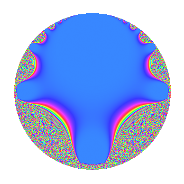This is a plot on a disk model. To make sense of this plot, I note that the real axis in the upper-half-plane model is the circumference of the circle, and the imaginary axis in the upper-half-plane model is the vertical diameter of the circle. In particular, $z = 0$ is the bottom of the circle, $z = i$ is the center of the circle, and $z = \infty$ is the top of the circle. The magnitude is currently displayed — the big blue region is where the magnitude is very small. In a neighborhood of the blue blob, there are a few bands of color that are meaningful — but then things change too quickly and the graph becomes a graph of noise.

I propose one of the following alternatives. I maintain the same badge and model for the space, but I change what is plotted and what colors to use. Also, I plot them larger so that we can get a good look at them; for the LMFDB they would probably be produced at the same (small) size.

## Plots with “Contours”

I have made three plots with contours. They are all morally the same, except for the underlying colorscheme. The “default” sage colorscheme leads to the following plot.The good thing is that it’s visually striking. But I recently learned that this colorscheme is hated, and it’s widely thought to be a poor choice in almost every situation.

A little bit ago, matplotlib added two colorschemes designed to fix the problems with the default colorscheme. (sage’s preferences are behind — the new matplotlib default has changed). This is one of them, called twilight.

##And this is the other default, called viridis. I don’t actually think this should be used, since the hues change from bright yellow to dark blue at complex-argument pi to negative pi. This gives the strong lines, which correspond to those places where the argument of the modular form is pi.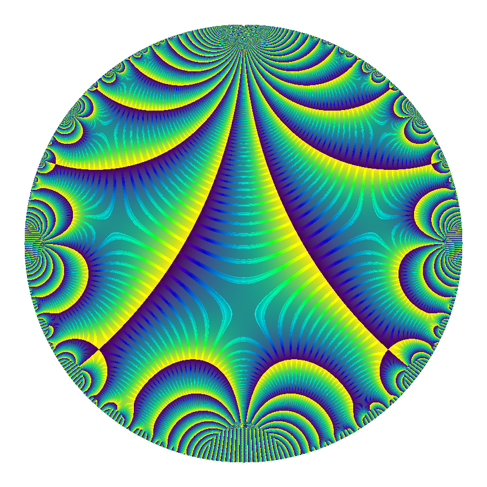Plots without Contours

I’ve also prepared these plots without the contours, and I think they’re quite nice as well.

First jet.Then twilight. At the talk I recently gave, this was the favorite — but I hadn’t yet implemented the contour-plots above for non-default colorschemes.Then viridis. (I’m still not serious about this one — but I think it’s pretty).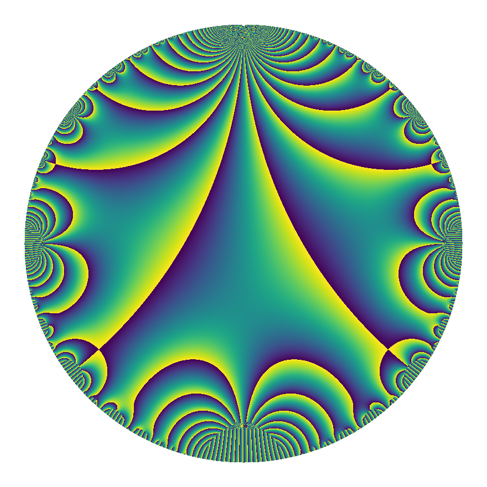Note on other Possibilities

There are other possibilities, such as perhaps plotting on a portion of the upper half-plane instead of a disk-model. I describe a few of these possibilities and give examples in the notes from my last talk. I should note that I can now produce contour-type plots there as well, though I haven’t done that.

For fun, here is the default colorscheme, but rotated. This came about accidentally (as did so many other plots in this excursion), but I think it highlights how odd jet is.

## Gathering Opinions

This concludes my proposal. I am collecting opinions. If you are struck by an idea or an opinion and would like to share it with me, please let me know, email me, or leave a comment below.

## Notes behind a talk: visualizing modular forms

Today, I’ll be at Bowdoin College giving a talk on visualizing modular forms. This is a talk about the actual process and choices involved in illustrating a modular form; it’s not about what little lies one might hold in their head in order to form some mental image of a modular form.1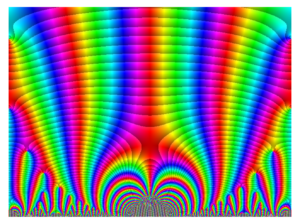This is a talk heavily inspired by the ICERM semester program on Illustrating Mathematics (currently wrapping up). In particular, I draw on2 conversations with Frank Farris (about using color to highlight desired features), Elias Wegert (about using logarithmically scaling contours), Ed Harriss (about the choice of colorscheme), and Brendan Hassett (about overall design choices).

There are very many pictures in the talk!

I wrote a few different complex-plotting routines for this project. At their core, they are based on sage’s complex_plot. There are two major variants that I use.

The first (currently called “ccomplex_plot”. Not a good name) overwrites how sage handles lightness in complex_plot in order to produce “contours” at spots where the magnitude is a two-power. These contours are actually a sudden jump in brightness.

The second (currently called “raw_complex_plot”, also not a good name) is even less formal. It vectorizes the computation and produces an object containing the magnitude and argument information for each pixel to be drawn. It then uses numpy and matplotlib to convert these magnitudes and phases into RGB colors according to a matplotlib-compatible colormap.

I am happy to send either of these pieces of code to anyone who wants to see them, but they are very much written for my own use at the moment. I intend to improve them for general use later, after I’ve experimented further.

In addition, I generated all the images for this talk in a single sagemath jupyter notebook (with the two .spyx cython dependencies I allude to above). This is also available here. (Note that using a service like nbviewer or nbconvert to view or convert it to html might be a reasonable idea).

As a final note, I’ll add that I mistyped several times in the preparation of the images for this talk. Included below are a few of the interesting-looking mistakes. The first two resulted from incorrectly applied conformal mappings, while the third came from incorrectly applied color correction.

Posted in Expository, Math.NT, Mathematics, sage, sagemath, sagemath | | 2 Comments

## Talk: Finding Congruent Numbers, Arithmetic Progressions of Squares, and Triangles

Here are some notes for my talk Finding Congruent Numbers, Arithmetic Progressions of Squares, and Triangles (an invitation to analytic number theory), which I’m giving on Tuesday 26 February at Macalester College.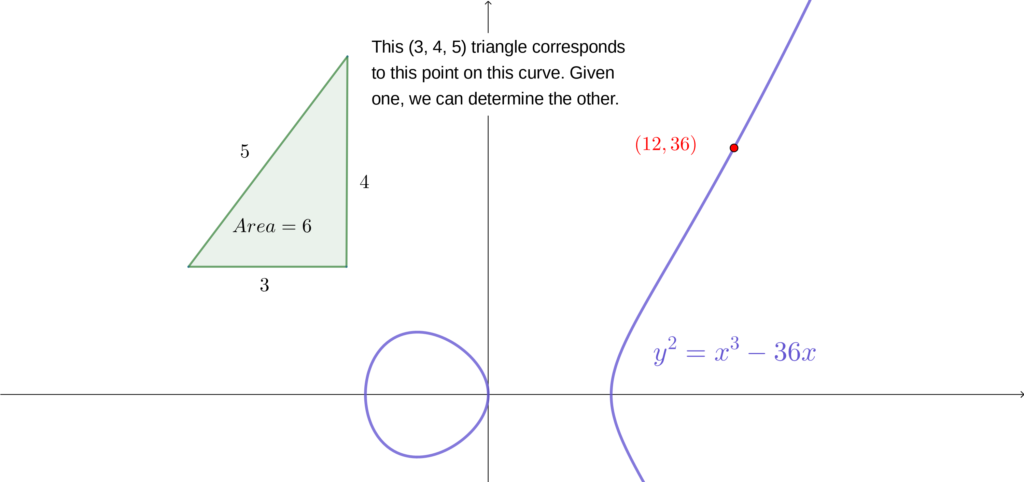The slides for my talk are available here.

The overarching idea of the talk is to explore the deep relationship between

1. right triangles with rational side lengths and area $n$,
2. three-term arithmetic progressions of squares with common difference $n$, and
3. rational points on the elliptic curve $Y^2 = X^3 – n^2 X$.

If one of these exist, then all three exist, and in fact there are one-to-one correspondences between each of them. Such an $n$ is called a congruent number.

By understanding this relationship, we also describe the ideas and results in the paper A Shifted Sum for the Congruent Number Problem, which I wrote jointly with Tom Hulse, Chan Ieong Kuan, and Alex Walker.

Towards the end of the talk, I say that in practice, the best way to decide if a (reasonably sized) number is congruent is through elliptic curves. Given a computer, we can investigate whether the number $n$ is congruent through a computer algebra system like sage.1

For the rest of this note, I’ll describe how one can use sage to determine whether a number is congruent, and how to use sage to add points on elliptic curves to generate more triangles corresponding to a particular congruent number.

Firstly, one needs access to sage. It’s free to install, but it’s quite large. The easiest way to begin using sage immediately is to use cocalc.com,  a free interface to sage (and other tools) that was created by William Stein, who also created sage.

In a sage session, we can create an elliptic curve through


> E6 = EllipticCurve([-36, 0])
> E6
Elliptic Curve defined by y^2 = x^3 - 36*x over Rational Field


More generally, to create the curve corresponding to whether or not $n$ is congruent, you can use


> n = 6   # (or anything you want)
> E = EllipticCurve([-n**2, 0])


We can ask sage whether our curve has many rational points by asking it to (try to) compute the rank.


> E6.rank()
1


If the rank is at least $1$, then there are infinitely many rational points on the curve and $n$ is a congruent number. If the rank is $0$, then $n$ is not congruent.2

For the curve $Y^2 = X^3 – 36 X$ corresponding to whether $6$ is congruent, sage returns that the rank is $1$. We can ask sage to try to find a rational point on the elliptic curve through


> E6.point_search(10)
[(-3 : 9 : 1)]


The 10 in this code is a limit on the complexity of the point. The precise definition isn’t important — using $10$ is a reasonable limit for us.

We see that this output something. When sage examines the elliptic curve, it uses the equation $Y^2 Z = X^3 – 36 X Z^2$ — it turns out that in many cases, it’s easier to perform computations when every term is a polynomial of the same degree. The coordinates it’s giving us are of the form $(X : Y : Z)$, which looks a bit odd. We can ask sage to return just the XY coordinates as well.


> Pt = E6.point_search(10)  # The  means to return the first element of the list
> Pt.xy()
(-3, 9)


In my talk, I describe a correspondence between points on elliptic curves and rational right triangles. In the talk, it arises as the choice of coordinates. But what matters for us right now is that the correspondence taking a point $(x, y)$ on an elliptic curve to a triangle $(a, b, c)$ is given by
$$(x, y) \mapsto \Big( \frac{n^2-x^2}{y}, \frac{-2 \cdot x \cdot y}{y}, \frac{n^2 + x^2}{y} \Big).$$

We can write a sage function to perform this map for us, through


> def pt_to_triangle(P):
x, y = P.xy()
return (36 - x**2)/y, (-2*x*6/y), (36+x**2)/y

> pt_to_triangle(Pt)
(3, 4, 5)


This returns the $(3, 4, 5)$ triangle!

Of course, we knew this triangle the whole time. But we can use sage to get more points. A very cool fact is that rational points on elliptic curves form a group under a sort of addition — we can add points on elliptic curves together and get more rational points. Sage is very happy to perform this addition for us, and then to see what triangle results.


> Pt2 = Pt + Pt
> Pt2.xy()
(25/4, -35/8)
> pt_to_triangle(Pt2)
(7/10, 120/7, -1201/70)


Another rational triangle with area $6$ is the $(7/10, 120/7, 1201/70)$ triangle. (You might notice that sage returned a negative hypotenuse, but it’s the absolute values that matter for the area). After scaling this to an integer triangle, we get the integer right triangle $(49, 1200, 1201)$ (and we can check that the squarefree part of the area is $6$).

Let’s do one more.


> Pt3 = Pt + Pt + Pt
> Pt3.xy()
(-1587/1369, -321057/50653)
> pt_to_triangle(Pt3)
(-4653/851, -3404/1551, -7776485/1319901)


That’s a complicated triangle! It may be fun to experiment some more — the triangles rapidly become very, very complicated. In fact, it was very important to the main result of our paper that these triangles become so complicated so quickly!

## Using lcalc to compute half-integral weight L-functions

This is a brief note intended primarily for my collaborators interested in using Rubinstein’s lcalc to compute the values of half-integral weight $L$-functions.

We will be using lcalc through sage. Unfortunately, we are going to be using some functionality which sage doesn’t expose particularly nicely, so it will feel a bit silly. Nonetheless, using sage’s distribution will prevent us from needing to compile it on our own (and there are a few bugfixes present in sage’s version).

Some $L$-functions are inbuilt into lcalc, but not half-integral weight $L$-functions. So it will be necessary to create a datafile containing the data that lcalc will use to generate its approximations. In short, this datafile will describe the shape of the functional equation and give a list of coefficients for lcalc to use.

## Building the datafile

It is assumed that the $L$-function is normalized in such a way that
$$\begin{equation} \Lambda(s) = Q^s L(s) \prod_{j = 1}^{A} \Gamma(\gamma_j s + \lambda_j) = \omega \overline{\Lambda(1 – \overline{s})}. \end{equation}$$
This involves normalizing the functional equation to be of shape $s \mapsto 1-s$. Also note that $Q$ will be given as a real number.

An annotated version of the datafile you should create looks like this

2                  # 2 means the Dirichlet coefficients are reals
0                  # 0 means the L-function isn't a "nice" one
10000              # 10000 coefficients will be provided
0                  # 0 means the coefficients are not periodic
1                  # num Gamma factors of form \Gamma(\gamma s + \lambda)
1                  # the \gamma in the Gamma factor
1.75 0             # \lambda in Gamma factor; complex valued, space delimited
0.318309886183790  # Q. In this case, 1/pi
1 0                # real and imaginary parts of omega, sign of func. eq.
0                  # number of poles
1.000000000000000  # a(1)
-1.78381067250408  # a(2)
...                # ...
-0.622124724090625 # a(10000)


If there is an error, lcalc will usually fail silently. (Bummer). Note that in practice, datafiles should only contain numbers and should not contain comments. This annotated version is for convenience, not for use.

You can find a copy of the datafile for the unique half-integral weight cusp form of weight $9/2$ on $\Gamma_0(4)$ here. This uses the first 10000 coefficients — it’s surely possible to use more, but this was the test-setup that I first set up.

## Generating the coefficients for this example

In order to create datafiles for other cuspforms, it is necessary to compute the coefficients (presumably using magma or sage) and then to populate a datafile. A good exercise would be to recreate this datafile using sage-like methods.

One way to create this datafile is to explicitly create the q-expansion of the modular form, if we happen to know a convenient expression. For us, we happen to know that $f = \eta(2z)^{12} \theta(z)^{-3}$. Thus one way to create the coefficients is to do something like the following.

num_coeffs = 10**5 + 1
weight     = 9.0 / 2.0

R.<q> = PowerSeriesRing(ZZ)
theta_expansion = theta_qexp(num_coeffs)
# Note that qexp_eta omits the q^(1/24) factor
eta_expansion = qexp_eta(ZZ[['q']], num_coeffs + 1)
eta2_coeffs = []
for i in range(num_coeffs):
if i % 2 == 1:
eta2_coeffs.append(0)
else:
eta2_coeffs.append(eta_expansion[i//2])
eta2 = R(eta2_coeffs)
g = q * ( (eta2)**4 / (theta_expansion) )**3

coefficients = g.list()[1:] # skip the 0 coeff
print(coefficients[:10])

normalized_coefficients = []
for idx, elem in enumerate(coefficients, 1):
normalized_coeff = 1.0 * elem / (idx ** (.5 * (weight - 1)))
normalized_coefficients.append(normalized_coeff)
print(normalized_coefficients[:10])


## Using lcalc now

Suppose that you have a datafile, called g1_lcalcfile.txt (for example). Then to use this from sage, you point lcalc within sage to this file. This can be done through calls such as

# Computes L(0.5 + 0i, f)
lcalc('-v -x0.5 -y0 -Fg1_lcalcfile.txt')

# Computes L(s, f) from 0.5 to (2 + 7i) at 1000 equally spaced samples
lcalc('--value-line-segment -x0.5 -y0 -X2 -Y7 --number-samples=1000 -Fg1_lcalcfile.txt')

# See lcalc.help() for more on calling lcalc.


The key in these is to pass along the datafile through the -F argument.

## A Jupyter Notebook from a SageMath tutorial

I gave an introduction to sage tutorial at the University of Warwick Computational Group seminar today, 2 November 2017. Below is a conversion of the sage/jupyter notebook I based the rest of the tutorial on. I said many things which are not included in the notebook, and during the seminar we added a few additional examples and took extra consideration to a few different calls. But for reference, the notebook is here.

The notebook itself (as a jupyter notebook) can be found and viewed on my github (link to jupyter notebook). When written, this notebook used a Sage 8.0.0.rc1 backend kernel and ran fine on the standard Sage 8.0 release , though I expect it to work fine with any recent official version of sage. The last cell requires an active notebook to be seen (or some way to export jupyter widgets to standalone javascript or something; this either doesn’t yet exist, or I am not aware of it).

I will also note that I converted the notebook for display on this website using jupyter’s nbconvert package. I have some CSS and syntax coloring set up that affects the display.

Good luck learning sage, and happy hacking.

# Sage¶

Sage (also known as SageMath) is a general purpose computer algebra system written on top of the python language. In Mathematica, Magma, and Maple, one writes code in the mathematica-language, the magma-language, or the maple-language. Sage is python.

But no python background is necessary for the rest of today’s guided tutorial. The purpose of today’s tutorial is to give an indication about how one really uses sage, and what might be available to you if you want to try it out.

I will spoil the surprise by telling you upfront the two main points I hope you’ll take away from this tutorial.

1. With tab-completion and documentation, you can do many things in sage without ever having done them before.
2. The ecosystem of libraries and functionality available in sage is tremendous, and (usually) pretty easy to use.

## Lightning Preview¶

Let’s first get a small feel for sage by seeing some standard operations and what typical use looks like through a series of trivial, mostly unconnected examples.

In :
# Fundamental manipulations work as you hope

2+3

Out:
5

You can also subtract, multiply, divide, exponentiate…

>>> 3-2
1
>>> 2*3
6
>>> 2^3
8
>>> 2**3 # (also exponentiation)
8



There is an order of operations, but these things work pretty much as you want them to work. You might try out several different operations.

Sage includes a lot of functionality, too. For instance,

In :
factor(-1008)

Out:
-1 * 2^4 * 3^2 * 7
In :
list(factor(1008))

Out:
[(2, 4), (3, 2), (7, 1)]

In the background, Sage is actually calling on pari/GP to do this factorization. Sage bundles lots of free and open source math software within it (which is why it’s so large), and provides a common access point. The great thing here is that you can often use sage without needing to know much pari/GP (or other software).

Sage knows many functions and constants, and these are accessible.

Posted in Expository, Mathematics, sage, sagemath | Tagged , , , , , | Leave a comment

## Introduction

The primary purpose of this note is to collect a few hitherto unnoticed or unpublished results concerning gaps between powers of consecutive primes. The study of gaps between primes has attracted many mathematicians and led to many deep realizations in number theory. The literature is full of conjectures, both open and closed, concerning the nature of primes.

In a series of stunning developments, Zhang, Maynard, and Tao12 made the first major progress towards proving the prime $k$-tuple conjecture, and successfully proved the existence of infinitely many pairs of primes differing by a fixed number. As of now, the best known result is due to the massive collaborative Polymath8 project,3 which showed that there are infinitely many pairs of primes of the form $p, p+246$. In the excellent expository article, 4 Granville describes the history and ideas leading to this breakthrough, and also discusses some of the potential impact of the results. This note should be thought of as a few more results following from the ideas of Zhang, Maynard, Tao, and the Polymath8 project.

Throughout, $p_n$ will refer to the $n$th prime number. In a paper, 5 Andrica conjectured that
\begin{equation}\label{eq:Andrica_conj}
\sqrt{p_{n+1}} – \sqrt{p_n} < 1
\end{equation}
holds for all $n$. This conjecture, and related statements, is described in Guy’s Unsolved Problems in Number Theory.
6 It is quickly checked that this holds for primes up to $4.26 \cdot 10^{8}$ in sagemath

# Sage version 8.0.rc1
# started with sage -ipython

# sage has pari/GP, which can generate primes super quickly
from sage.all import primes_first_n

# import izip since we'll be zipping a huge list, and sage uses python2 which has
# non-iterable zip by default
from itertools import izip

# The magic number 23150000 appears because pari/GP can't compute
# primes above 436273290 due to fixed precision arithmetic
ps = primes_first_n(23150000)    # This is every prime up to 436006979

# Verify Andrica's Conjecture for all prime pairs = up to 436006979
gap = 0
for a,b in izip(ps[:-1], ps[1:]):
if b**.5 - a**.5 > gap:
A, B, gap = a, b, b**.5 - a**.5
print(gap)
print("")
print(A)
print(B)


In approximately 20 seconds on my machine (so it would not be harder to go much higher, except that I would have to go beyond pari/GP to generate primes), this completes and prints out the following output.

0.317837245196
0.504017169931
0.670873479291

7
11


Thus the largest value of $\sqrt{p_{n+1}} – \sqrt{p_n}$ was merely $0.670\ldots$, and occurred on the gap between $7$ and $11$.

So it appears very likely that the conjecture is true. However it is also likely that new, novel ideas are necessary before the conjecture is decided.

Andrica’s Conjecture can also be stated in terms of prime gaps. Let $g_n = p_{n+1} – p_n$ be the gap between the $n$th prime and the $(n+1)$st prime. Then Andrica’s Conjecture is equivalent to the claim that $g_n < 2 \sqrt{p_n} + 1$. In this direction, the best known result is due to Baker, Harman, and Pintz, 7 who show that $g_n \ll p_n^{0.525}$.

In 1985, Sandor 8 proved that \begin{equation}\label{eq:Sandor} \liminf_{n \to \infty} \sqrt{p_n} (\sqrt{p_{n+1}} – \sqrt{p_n}) = 0. \end{equation} The close relation to Andrica’s Conjecture \eqref{eq:Andrica_conj} is clear. The first result of this note is to strengthen this result.

Theorem

Let $\alpha, \beta \geq 0$, and $\alpha + \beta < 1$. Then
\begin{equation}\label{eq:main}
\liminf_{n \to \infty} p_n^\beta (p_{n+1}^\alpha – p_n^\alpha) = 0.
\end{equation}

We prove this theorem below. Choosing $\alpha = \frac{1}{2}, \beta = \frac{1}{4}$ verifies Sandor’s result \eqref{eq:Sandor}. But choosing $\alpha = \frac{1}{2}, \beta = \frac{1}{2} – \epsilon$ for a small $\epsilon > 0$ gives stronger results.

This theorem leads naturally to the following conjecture.

Conjecture

For any $0 \leq \alpha < 1$, there exists a constant $C(\alpha)$ such that
\begin{equation}
p_{n+1}^\alpha – p_{n}^\alpha \leq C(\alpha)
\end{equation}
for all $n$.

A simple heuristic argument, given in the last section below, shows that this Conjecture follows from Cramer’s Conjecture.

It is interesting to note that there are generalizations of Andrica’s Conjecture. One can ask what the smallest $\gamma$ is such that
\begin{equation}
p_{n+1}^{\gamma} – p_n^{\gamma} = 1
\end{equation}
has a solution. This is known as the Smarandache Conjecture, and it is believed that the smallest such $\gamma$ is approximately
\begin{equation}
\gamma \approx 0.5671481302539\ldots
\end{equation}
The digits of this constant, sometimes called “the Smarandache constant,” are the contents of sequence A038458 on the OEIS. It is possible to generalize this question as well.

Open Question

For any fixed constant $C$, what is the smallest $\alpha = \alpha(C)$ such that
\begin{equation}
p_{n+1}^\alpha – p_n^\alpha = C
\end{equation}
has solutions? In particular, how does $\alpha(C)$ behave as a function of $C$?

This question does not seem to have been approached in any sort of generality, aside from the case when $C = 1$.

## Proof of Theorem

The idea of the proof is very straightforward. We estimate \eqref{eq:main} across prime pairs $p, p+246$, relying on the recent proof from Polymath8 that infinitely many such primes exist.

Fix $\alpha, \beta \geq 0$ with $\alpha + \beta < 1$. Applying the mean value theorem of calculus on the function $x \mapsto x^\alpha$ shows that
\begin{align}
p^\beta \big( (p+246)^\alpha – p^\alpha \big) &= p^\beta \cdot 246 \alpha q^{\alpha – 1} \\\
&\leq p^\beta \cdot 246 \alpha p^{\alpha – 1} = 246 \alpha p^{\alpha + \beta – 1}, \label{eq:bound}
\end{align}
for some $q \in [p, p+246]$. Passing to the inequality in the second line is done by realizing that $q^{\alpha – 1}$ is a decreasing function in $q$. As $\alpha + \beta – 1 < 0$, as $p \to \infty$ we see that\eqref{eq:bound} goes to zero.

Therefore
\begin{equation}
\liminf_{n \to \infty} p_n^\beta (p_{n+1}^\alpha – p_n^\alpha) = 0,
\end{equation}
as was to be proved.

## Further Heuristics

Cramer’s Conjecture states that there exists a constant $C$ such that for all sufficiently large $n$,
\begin{equation}
p_{n+1} – p_n < C(\log n)^2.
\end{equation}
Thus for a sufficiently large prime $p$, the subsequent prime is at most $p + C (\log p)^2$. Performing a similar estimation as above shows that
\begin{equation}
(p + C (\log p)^2)^\alpha – p^\alpha \leq C (\log p)^2 \alpha p^{\alpha – 1} =
C \alpha \frac{(\log p)^2}{p^{1 – \alpha}}.
\end{equation}
As the right hand side vanishes as $p \to \infty$, we see that it is natural to expect that the main Conjecture above is true. More generally, we should expect the following, stronger conjecture.

Conjecture’

For any $\alpha, \beta \geq 0$ with $\alpha + \beta < 1$, there exists a constant $C(\alpha, \beta)$ such that
\begin{equation}
p_n^\beta (p_{n+1}^\alpha – p_n^\alpha) \leq C(\alpha, \beta).
\end{equation}